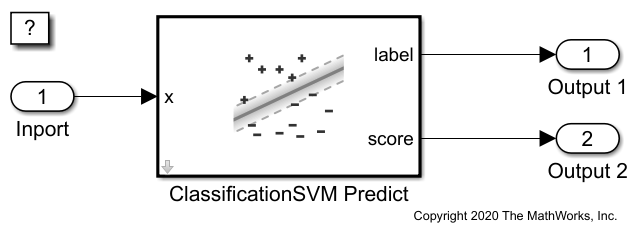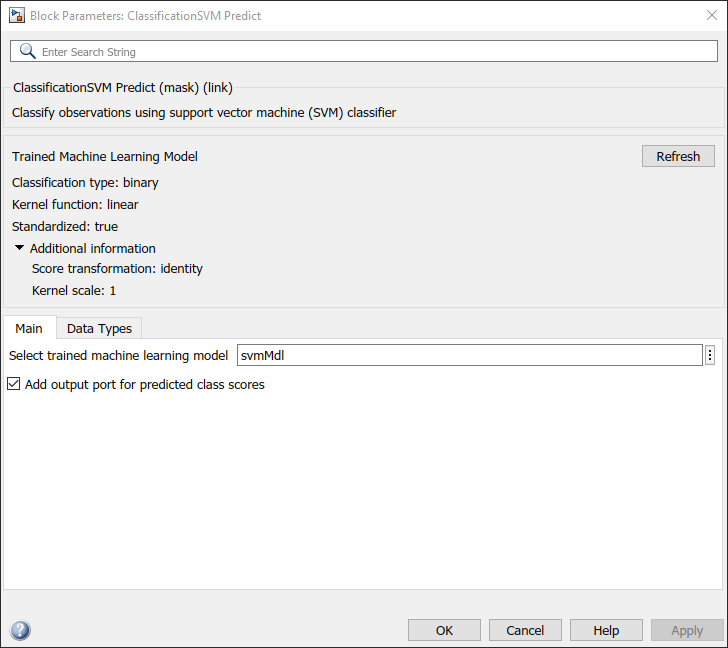# Predict Class Labels Using ClassificationSVM Predict Block

This example shows how to use the ClassificationSVM Predict block for label prediction. The block accepts an observation (predictor data) and returns the predicted class label and class score for the observation using a trained support vector machine (SVM) classification model.

### Train Classification Model

This example uses the `ionosphere` data set, which contains radar return qualities (`Y`) and predictor data (`X`) of 34 variables. Radar returns are either of good quality (`'g'`) or bad quality (`'b'`).

Load the `ionosphere` data set. Determine the sample size.

```load ionosphere n = numel(Y)```
```n = 351 ```

Suppose that the radar returns are detected in sequence, and you have the first 300 observations, but you have not received the last 51 yet. Partition the data into present and future samples.

```prsntX = X(1:300,:); prsntY = Y(1:300); ftrX = X(301:end,:); ftrY = Y(301:end);```

Train an SVM model using all presently available data. Specify predictor data standardization.

`svmMdl = fitcsvm(prsntX,prsntY,'Standardize',true);`

`svmMdl` is a `ClassificationSVM` model.

Check the negative and positive class names by using the `ClassNames` property of `svmMdl`.

`svmMdl.ClassNames`
```ans = 2×1 cell {'b'} {'g'} ```

The negative class is `'b'`, and the positive class is `'g'`. The output values from the Score port of the ClassificationSVM Predict block have the same order. The first and second elements correspond to the negative class and positive class scores, respectively.

This example provides the Simulink model `slexIonosphereClassificationSVMPredictExample.slx`, which includes the ClassificationSVM Predict block. You can open the Simulink model or create a new model as described in this section.

Open the Simulink model `slexIonosphereClassificationSVMPredictExample.slx`.

```SimMdlName = 'slexIonosphereClassificationSVMPredictExample'; open_system(SimMdlName)```The `PreLoadFcn` callback function of `slexIonosphereClassificationSVMPredictExample` includes code to load the sample data, train the SVM model, and create an input signal for the Simulink model. If you open the Simulink model, then the software runs the code in `PreLoadFcn` before loading the Simulink model. To view the callback function, on the Modeling tab, in the Setup section, select Model Settings > Model Properties. Then, on the Callbacks tab, select the `PreLoadFcn` callback function in the Model callbacks pane.

To create a new Simulink model, open the Blank Model template and add the ClassificationSVM Predict block. Add the Inport and Outport blocks and connect them to the ClassificationSVM Predict block.

Double-click the ClassificationSVM Predict block to open the Block Parameters dialog box. Specify the Select trained machine learning model parameter as `svmMdl`, which is the name of a workspace variable that contains the trained SVM model. Click the action button (with three vertical dots) or click Update Model on the Modeling tab. The dialog box displays the classification type and the options used to train the SVM model `svmMdl` under Trained Machine Learning Model. Select the Add output port for predicted class scores check box to add the second output port Score.The ClassificationSVM Predict block expects an observation containing 34 predictor values. Double-click the inport block, and set the Port dimensions to 34 on the Signal Attributes tab.

Create an input signal data in the form of a structure array for the Simulink model. The structure array must contain these fields:

• `time` — The points in time at which the observations enter the model. In this example, the duration includes the integers from 0 through 50. The orientation must correspond to the observations in the predictor data. So, in this case, `time` must be a column vector.

• `signals` — A 1-by-1 structure array describing the input data and containing the fields `values` and `dimensions`, where `values` is a matrix of predictor data, and `dimensions` is the number of predictor variables.

Create an appropriate structure array for future radar returns.

```radarReturnInput.time = (0:50)'; radarReturnInput.signals(1).values = ftrX; radarReturnInput.signals(1).dimensions = size(ftrX,2);```

To import signal data from the workspace:

• Open the Configuration Parameters dialog box. On the Modeling tab, click Model Settings.

• In the Data Import/Export pane, select the Input check box and enter `carsmallInput` in the adjacent text box.

• In the Solver pane, under Simulation time, set Stop time to `radarReturnInput.time(end)`. Under Solver selection, set Type to `Fixed-step`, and set Solver to `discrete (no continuous states)`.

Simulate the model.

`sim(SimMdlName);`

When the inport block detects an observation, it directs the observation into the ClassificationSVM Predict block. You can use the Simulation Data Inspector (Simulink) to view the logged data of the Outport blocks.